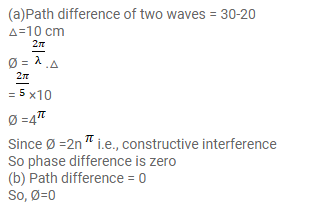# Two point sources of sound are kept at a separation of`
Question:

Two point sources of sound are kept at a separation of $10 \mathrm{~cm}$. They vibrate in phase to produce waves of wavelength $5.0 \mathrm{~cm}$. What would be the phase difference between the two waves arriving at a point $20 \mathrm{~cm}$ from one source (a) on the line joining the sources and (b) on the perpendicular bisector of the line joining the sources?

Solution: